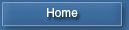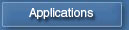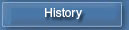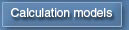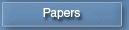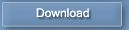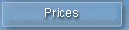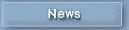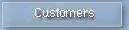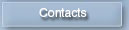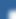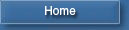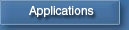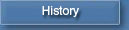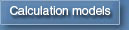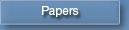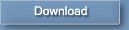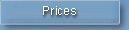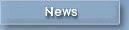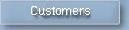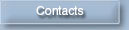Diesel-RK in Telegram## Multiparametrical optimization

While solving research problems connected to searching optimal combinations of several engine parameters, such as compression ratio, injection timing, diameter, number and direction of injector nuzzles, combustion chamber shape, valve timing, turbocharging parameters etc., it can be hard to plan and treat with a numerical experiment with many variable factors. Very effective here is multiparametrical optimization, when searching of optimal combination of variable factors is laid on the formal procedure of non-linear programming, and the researcher only has to formulate the optimal search problem correctly and analyze obtained solution. Optimal search procedures during searching an extremum of a multiparametrical function use the super- or turbocharged engine mathematical model to connect independent variables (optimization parameters) with the goal function and with restrictions.

Goal function

Parameters of efficiency of an engine or its separate processes can be included in a goal function:

Zj = Zj (Xk).

The finding of an extremum of a goal function is a problem of optimization.

Vector of explanatory variables

Vector of explanatory variables Xk is a set of design data of an engine. At the expense of selection of value of these data is planned to achieve an extremum of a goal function.

Restrictions

As a rule at a searching of optimum combination of  ICE design data it is necessary to monitor engine thermal and mechanical tensions, emission level  and other monitoring factors which limit area of optimum searching and are the restrictions. The restraining parameters, as well as a goal function depend on explanatory variables:

Yi = Yi (Xk).

The analytical relation between a goal function both restrictions on the one hand and vector of explanatory variables on the other hand does not exist, therefore for their calculation the mathematical model of   ICE is used.

Thus, the problem of optimization of an engine is reduced to a problem of non-linear programming, i.e. to a problem of searching of an optimum of a function Zj (Xk) at presence of limitations: Yi min < Yi (Xk) < Y i max.

The presence of restrictions essentially complicates a solution of optimization problems, therefore it is expedient to reduce a problem of conditional optimization to a problem of non-conditional optimization, the algorithms for which much better designed. Method of penal functions is an effective mode to take into account of restrictions. The essence of a method is, that at violation of restriction to a minimized goal function the penalty is added. The penalty will increase in accordance with magnification of violation of restriction. Generally the goal function is a sum of three items. Each item has its influence coefficient Czj, Cyi, Cxk.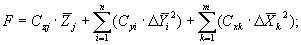where: Czj is a line factor (influence coefficient) of optimized ICE parameter Zj included into goal function;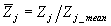is a relative ICE parameter Zj related to its mean average value (for example: sfc, power, NO emission, etc.);
 Cyi is a penal factor for leaving permitted area of  Yi;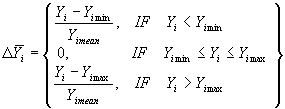is a related value of violation of restriction Yi;
 Cxk is a penal factor for leaving permitted area of Xk ;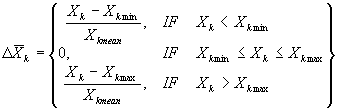is a related value of Xk lapped over allowable borders;

Mean values of explanatory variables Xk mean   and restrictions Yi mean as well as penal factors of Xk  are set by program.
Specification of penal factors for restrictions Cyi ;   max. and min. borders for restrictions Yi minYi max and explanatory variables Xi minXi max as well as goal function Zj has to be made by user in preprocessor of DIESEL-RK.

Library of algorithms of  DIESEL-RK  software

For searching an extremum of function of many variables the well known algorithms of nonlinear programming are used.

Non-linear programming theory doesn't say which methods are better so while selecting these methods the researcher should be guided by his own experience of optimization problems salvation.

DIESEL-RK library contains 14 algorithms:

Zero-order methods don't use partial derivatives of goal function:

• On-coordinates descent method.
• "Deformable polyhedron" method by Nelder and Mead.
• Rosenbrok method.
• Powell method.

First-order methods use partial derivatives of goal function:

• "Quickest descent" method.
• "Heavy ball" method.
• Flatcher-Reeves method.
• Polak-Ribier method.
• Projective method of Newton-Rafson.
• Davidon-Flatcher-Powell method.
• Broiden method (rank 1).
• Pearson method 2.
• Pearson method 3.

Random search method:
Monte-Carlo method.

All algorithms provide finding solutions of optimization problems with different efficiency. DIESEL-RK developers can only advise to use first-order methods in the cases when expected optimum is far from the starting point. Methods of quickest descent and heavy ball also give good results.

The great many of methods is necessary for checkout of the obtained solutions. If the solutions obtained by different methods are similar, there is greater reliance that the obtained solution is really optimal.

Usage of the optimization program is especially effective at solving the problems of engine boosting, at development of new constructions, and also at modernization directed on decrease of fuel consumption and emission of harmful materials.

Example of usage of the optimization is represented in page: Multiparametric optimization of a medium-speed marine diesel at increase its capacity.

Bibliography

1. Hooke R., Jeeves T.A. Direct Search Solution of Numerical and Statistical Problems. S. Assoc. Computer. Mach. 8,1962, pp.212- 229.
2. Nelder J.A., Mead R. Computer J., 7, 1964, pp.368.
3. Rosenbrok H.H. Computer J., 3,1960, pp.175.
4. Powell M.J.D. Computer J., 7,1964, pp.155; 7,1965, pp.303.
5. Pearson J.D. Computer J., 13,1969, pp.171.
6. Flatcher R., Powell M.J.D. Computer J., 6,1963, pp.163-168.
7. Flatcher R., Reeves C.M. Computer J., 7,1964, pp.149.

### Scanning

If an optimization problem can be formulated as a 2D-problem (number of variables = 2), it is optimal to use scanning, when engine parameters are calculated in the nods of an orthogonal grid, where X-axis is used for the first parameter and Y-axis - for the second parameter. Scanning results are used to build surfaces, where many parameters can be displayed in form which can be used for analysis:
- 3D graph of a function of two arguments,
- isoline family (sections of these surfaces at different function levels).

Example: 2D scanning results are displayed as surface plot and isoline families.
SFC and volumetric efficiency of four-stroke diesel as functions of gas exchange timing:   EVC and IVO.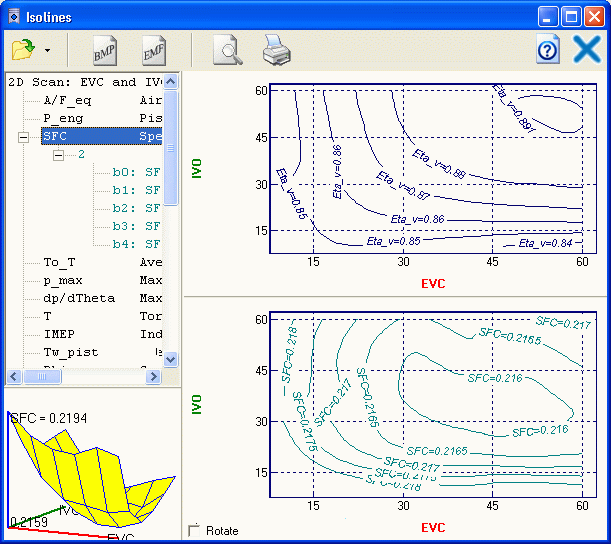Enlarged 3D diagram.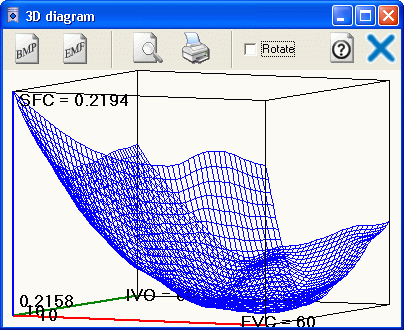Use of the scanning allows fast finding the effective decisions at development of engines.